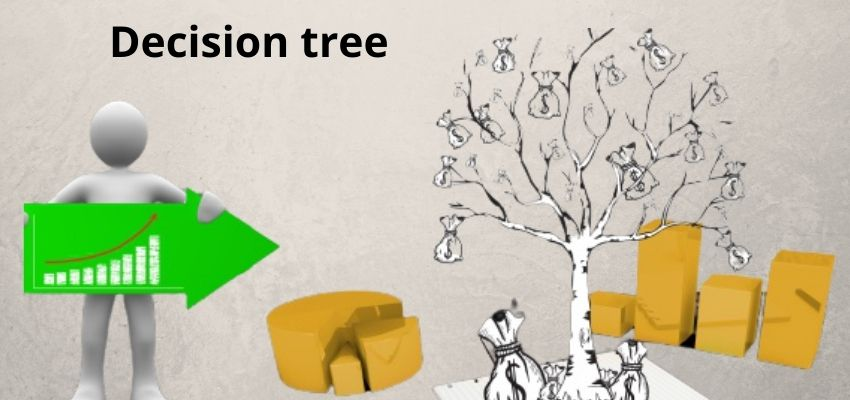# What Is a Decision Tree?A decision tree is a predictive tree, which shows the various types of results from a series of conditionals. It helps us to take the decision from the serious of outcomes. We can make a decision tree is in the form of a flowchart. We can use a decision tree as a decision-making tool for the problem where we need series of outcomes, this also helps us to analyze the researcher’s work by seeing the problem in various ways and analyze the problem in series of ways. If you are planning something then a decision tree will help you in this also. It will show the predictive result of your planning ad which will help you to make a decision whether it’s profitable or not.

A decision tree has a flowchart-like structure, which easy to make and understand. It is similar to a tree. Where each internal node or part signifies a test of the attribute and each branch shows the outcome of the test, and every leaf holds a class of the tree. We can call leaf as a terminal node that holds the class label.

## Detailing of Decision Tree Nodes

• Root Node

It represents the actual problem is or we can say it a sample of the problem where everything is specified just only the problem details not the solution and then it is further gets divided into two or more similar types of problems.

• Splitting

In this, we divide the node into two or more parts. In this, your problem is divided into a more predictive node which helps us to make decisions.

• Decision Node

When the divided sub-node further divided into other sub-nodes, then it is called the decision node. When your problem is divided into different conditions then a decision node is created.

• Leaf / Terminal Node

Which Nodes further do not expand or divide then is called Leaf or Terminal node. When your conditional problem shows the result then this is known as a leaf node.

• Pruning

When we remove sub-nodes of a decision node that does not require any more, this process is called pruning. This process is the opposite of splitting where we division the nodes.

• Branch / Sub-Tree

A subsection or the conditional state of the entire tree or problem is called a branch or sub-tree.

• Parent and Child Node

A node or a problem, which is split into conditional states or sub-nodes is called a parent node. Each sub-node is called the child node of the parent node.

### Pros of Decision Tree

• It is easy and simple to understand
• We can easily draw and translate the complex problem
• It gives you an Expert opinion
• We can include preferences also otherwise it will be hard data to understand.
• We can compare the problem with other decision techniques and methods.
• New scenarios or nodes can easily be added and expanded.

### Cons of Decision Tree

• When you need to take a decision for a categorical variable where a number of categories are there, then it will be very complex and hard to understand because there is a large number of information levels are there.
• It is difficult to do quick calculations of the complex problem because this is only a problem and it is just a tree that is created by the hand. It is very difficult to calculate a complex problem in seconds.

## How to Draw a Decision Tree

You can draw it by hand on paper or a whiteboard, or you can use special decision tree software also to make a decision tree. These are the steps to follow:

Draw a small box that will represent the problem point in your tree and specify the starting point of the tree, then draw a line from the box to the right side which will represent all possible conditions and solutions. Label them according to your problem.